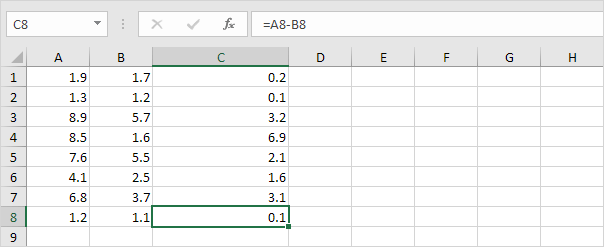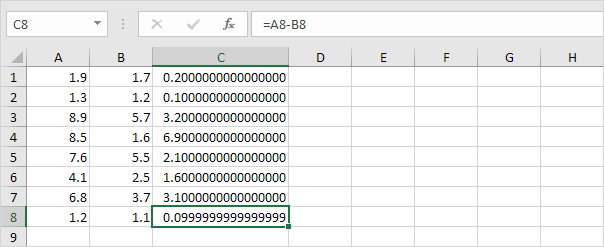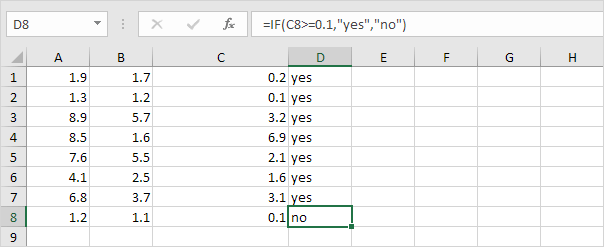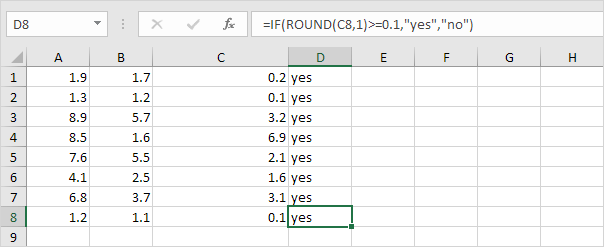# Floating Point Errors

Excel stores and calculates floating point numbers. Sometimes, the result of a formula is a very close approximation.

1. For example, take a look at the formulas below. At first glance, everything looks alright.2. However, if we show 16 decimal places, we can see that one result is a very close approximation.You don't have to worry about floating point errors. They are rare.

3. Even if your worksheet contains a floating point error, in most cases, this causes no problems. However, if you compare the value in cell C8 with another value, the following problem can occur.4. Use the ROUND function to fix this.Go to Next Chapter: Array Formulas